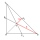# Internal angles

The ABCD is an isosceles trapezoid, which holds:
|AB| = 2 |BC| = 2 |CD| = 2 |DA|:

On its side BC is a K point such that |BK| = 2 |KC|, on its side CD is the point L such that |CL| = 2 |LD|, and on its side DA the point M is such that | DM | = 2 |MA|. Determine the internal angles of the KLM triangle.

Result

∠KLM =  120 °
∠LMK =  30 °
∠MKL =  30 °

Leave us a comment of example and its solution (i.e. if it is still somewhat unclear...):

Showing 1 comment:Math student
Help. First, look at the inner angles of the ABCD trapezoid.

Solution. It follows from the assumptions that the center line of the AB segment with the vertices C and D divides the ABCD trapezoid into three identical equilateral triangles. Therefore, the magnitude of internal angles in the trapezoid at A and B vertices is equal to 60 °
And at the C and D vertices 120 °. It follows from the specification that the triangles LCK and MDL are the same (according to the sentence above). Therefore, both the KL and LM lines and the designated pairs of angles are the same; The magnitudes of these angles are denoted α and β. The triangle KLM is isosceles and the angles at the base are the same; Their size is denoted by δ and the size of the angle KLM is denoted by γ.

From the sum of the inner angles in the KCL triangle we derive
α + β = 180° − 120° = 60°

The sum of the three marked angles with the vertex L is a straight angle, therefore
γ = 180° − (α + β) = 120°
Finally, we deduce the sum of inner angles in the triangle KLM
δ = (180° − 120°)/2 = 30°

The internal angles of the triangle KLM are 30° and 120°#### To solve this example are needed these knowledge from mathematics:

See also our trigonometric triangle calculator.

## Next similar examples:

1. Find the 9Find the missing angle in the triangle and then name triangle. Angles are: 95, 2x+15, x+3
2. Trapezoid MOThe rectangular trapezoid ABCD with right angle at point B, |AC| = 12, |CD| = 8, diagonals are perpendicular to each other. Calculate the perimeter and area of ​​the trapezoid.
3. Isosceles triangle 8If the rate of the sides an isosceles triangle is 7:6:7, find the base angle correct to the nearest degree.
4. LighthouseMarcel (point J) lies in the grass and sees the top of the tent (point T) and behind it the top of the lighthouse (P). | TT '| = 1.2m, | PP '| = 36m, | JT '| = 5m. Marcel lies 15 meters away from the sea (M). Calculate the lighthouse distance from the sea.
5. Triangle SASCalculate area and perimeter of the triangle, if the two sides are 51 cm and 110 cm long and angle them clamped is 130°.
6. Cable carFind the elevation difference of the cable car when it rises by 67 per mille and the rope length is 930 m.
7. Trigonometric functionsIn right triangle is: ? Determine the value of s and c: ? ?
8. The sides 2The sides of a trapezoid are in the ratio 2:5:8:5. The trapezoid’s area is 245. Find the height and the perimeter of the trapezoid.
9. Medians 2:1Median to side b (tb) in triangle ABC is 12 cm long. a. What is the distance of the center of gravity T from the vertex B? b, Find the distance between T and the side b.
10. GardenArea of square garden is 6/4 of triangle garden with sides 56 m, 35 m and 35 m. How many meters of fencing need to fence a square garden?
11. Tetrahedral pyramidWhat is the surface of a regular tetrahedral (four-sided) pyramid if the base edge a=7 and height v=6?
12. Find parametersFind parameters of the circle in the plane - coordinates of center and radius: ?
13. CincinnatiA map is placed on a coordinate grid. Cincinnati located at (5,4) and San Diego is located at (-10, -3). How far apart is Cincinnati from San Diego on the map? Round to the nearest tenth.
14. Right ΔRight triangle has length of one leg 28 cm and length of the hypotenuse 53 cm. Calculate the height of the triangle.
15. Axial sectionAxial section of the cone is equilateral triangle with area 208 dm2. Calculate volume of the cone.
16. Circle chordWhat is the length d of the chord circle of diameter 36 m, if distance from the center circle is 16 m?
17. RectangleThe rectangle is 11 cm long and 45 cm wide. Determine the radius of the circle circumscribing rectangle.